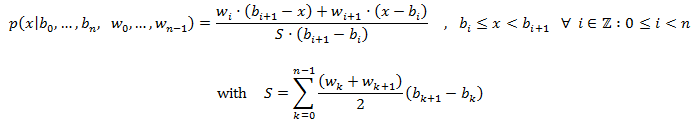class template
<random>

# std::piecewise_linear_distribution

`template <class RealType = double> class piecewise_linear_distribution;`
Piecewise linear distribution
Random number distribution that produces floating-point values that are distributed over a sequence of contiguous subintervals, of which the probability density at its boundaries is specified, as if defined by the following probability density function:A set of n non-negative individual weights (the w's) for each of the n subinterval bounds (bi) are set on construction. The probability density on the bounds is proportional to those weights in such a way that the integral that covers all possible values is exactly 1.0.
The probability of producing a value within each subinterval is the linear value in between the probability density of its bounds.

To produce a random value following this distribution, call its member function operator().

### Template parameters

RealType
A floating-point type. Aliased as member type result_type.
By default, this is double.

### Member types

The following aliases are member types of piecewise_linear_distribution:

member typedefinitionnotes
result_typeThe first template parameter (RealType)The type of the numbers generated (defaults to double)
param_typenot specifiedThe type returned by member param.

### Example

 ``123456789101112131415161718192021222324252627282930`` ``````// piecewise_linear_distribution #include #include #include int main() { const int nrolls = 10000; // number of experiments const int nstars = 100; // maximum number of stars to distribute std::default_random_engine generator; std::array intervals {0.0, 4.5, 9.0}; std::array weights {10.0, 0.0, 10.0}; std::piecewise_linear_distribution distribution (intervals.begin(),intervals.end(),weights.begin()); int p={}; for (int i=0; i

Possible output:
 ```a piecewise_linear_distribution: 0-1: ******************* 1-2: ************** 2-3: ********* 3-4: ***** 4-5: * 5-6: ***** 6-7: ********* 7-8: ************** 8-9: ******************* ```